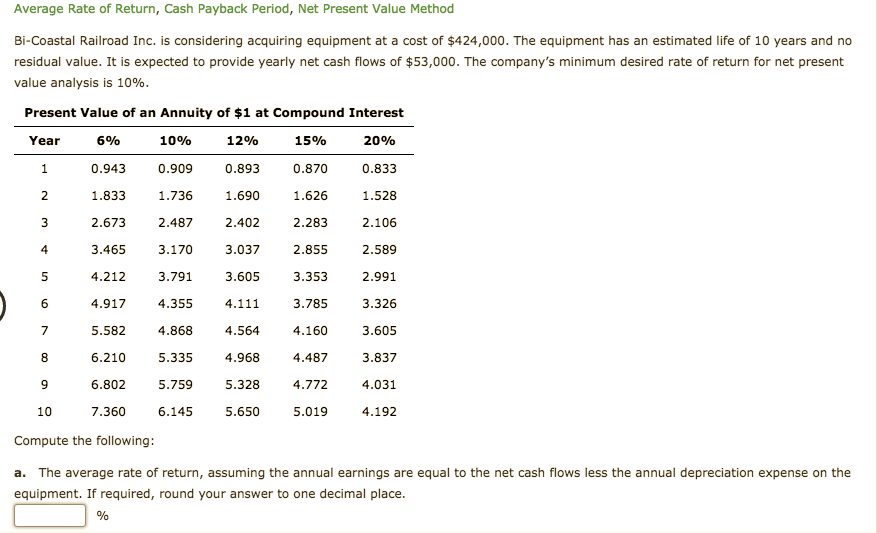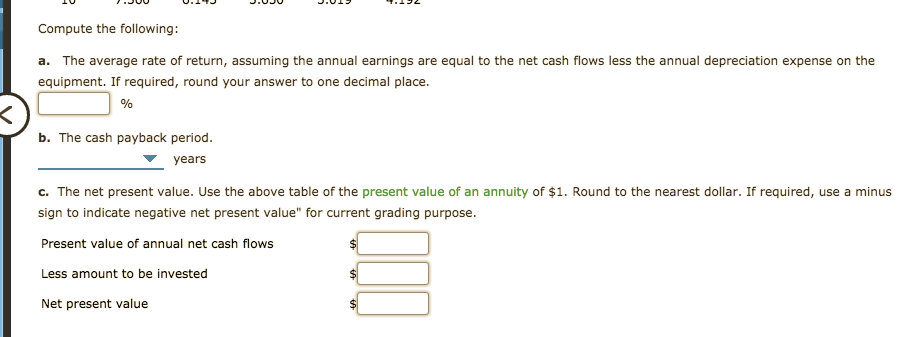# Average Rate of Return, Cash Payback Period, Net Present Value MethodBi-Coastal Railroad Inc. is considering acquiring equipment at a cost of \$424,000. The equipment has an estimated life of 10 years and noresidual value. It is expected to provide yearly net cash flows of \$53,000. The company's minimum desired rate of return for net presentvalue analysis is 10%.Present Value of an Annuity of \$1 at Compound InterestYear6%10%12%15%20%0.9430.9090.8930.8700.8331.8331.6901.7361.6261.5282.6732.4872.4022.2832.1063.4653.1703.0372.8552.5893.7912.9914.2123.6053.3534.9174.3554.1113.7853.3265.5824.8684.5644.1603.6056.2105.3354.9684.4873.8374.0316.8025.7595.3284.7726.1455.650107.3605.0194.192Compute the following:a. The average rate of return, assuming the annual earnings are equal to the net cash flows less the annual depreciation expense on theequipment. If required, round your answer to one decimal place. Compute the following:a. The average rate of return, assuming the annual earnings are equal to the net cash flows less the annual depreciation expense on theequipment. If required, round your answer to one decimal place.b. The cash payback period.yearsc. The net present value. Use the above table of the present value of an annuity of \$1. Round to the nearest dollar. If required, use a minussign to indicate negative net present value" for current grading purpose.Present value of annual net cash flowsLess amount to be investedNet present value

Question
34 viewshelp_outlineImage TranscriptioncloseAverage Rate of Return, Cash Payback Period, Net Present Value Method Bi-Coastal Railroad Inc. is considering acquiring equipment at a cost of \$424,000. The equipment has an estimated life of 10 years and no residual value. It is expected to provide yearly net cash flows of \$53,000. The company's minimum desired rate of return for net present value analysis is 10%. Present Value of an Annuity of \$1 at Compound Interest Year 6% 10% 12% 15% 20% 0.943 0.909 0.893 0.870 0.833 1.833 1.690 1.736 1.626 1.528 2.673 2.487 2.402 2.283 2.106 3.465 3.170 3.037 2.855 2.589 3.791 2.991 4.212 3.605 3.353 4.917 4.355 4.111 3.785 3.326 5.582 4.868 4.564 4.160 3.605 6.210 5.335 4.968 4.487 3.837 4.031 6.802 5.759 5.328 4.772 6.145 5.650 10 7.360 5.019 4.192 Compute the following: a. The average rate of return, assuming the annual earnings are equal to the net cash flows less the annual depreciation expense on the equipment. If required, round your answer to one decimal place. fullscreenhelp_outlineImage TranscriptioncloseCompute the following: a. The average rate of return, assuming the annual earnings are equal to the net cash flows less the annual depreciation expense on the equipment. If required, round your answer to one decimal place. b. The cash payback period. years c. The net present value. Use the above table of the present value of an annuity of \$1. Round to the nearest dollar. If required, use a minus sign to indicate negative net present value" for current grading purpose. Present value of annual net cash flows Less amount to be invested Net present value fullscreen
check_circle

star
star
star
star
star
1 Rating
Step 1

Net present value of a project is calculated by deducting the cost of project from the present value of cash inflows. A project is selected if it has positive net present value and vice-a-versa.

Payback period is the period of time in which the cost of project is covered from cash inflows of the project.

Step 2

a)

Cost of equipment: \$424,000

Useful life of equipment: 10 years

Salvage value: \$0

Depreciation expense per annum is calculated as follows:

Step 3

Annual earnings: net cash flows – annual depreciation expense

Annual earnings: \$53,000 - \$42,400 = \$10,600

A...

### Want to see the full answer?

See Solution

#### Want to see this answer and more?

Solutions are written by subject experts who are available 24/7. Questions are typically answered within 1 hour.*

See Solution
*Response times may vary by subject and question.
Tagged in